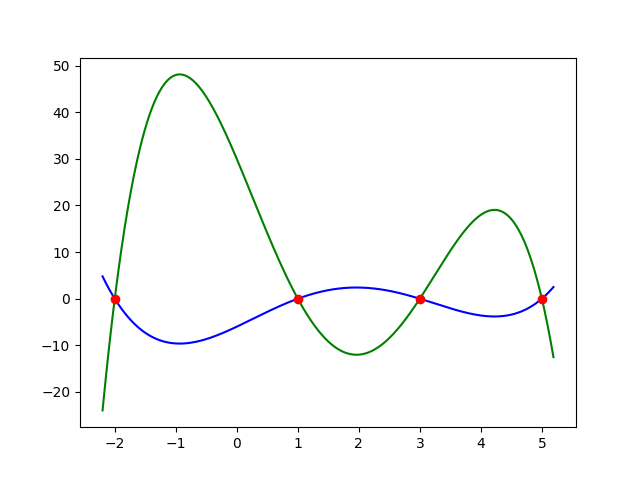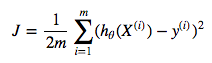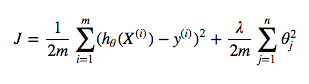HomeTutorialsMachine Learning

# Towards Preventing Overfitting in Machine Learning: Regularization

Learn the basics of Regularization and how it helps to prevent Overfitting.
Aug 2018  · 8 min read

In machine learning, you must have come across the term Overfitting. Overfitting is a phenomenon where a machine learning model models the training data too well but fails to perform well on the testing data. Performing sufficiently good on testing data is considered as a kind of ultimatum in machine learning.

There are quite a number of techniques which help to prevent overfitting. Regularization is one such technique. And this post is all dedicated to that. In this post, I will try to explain Regularization with less math but with more intuition. Unlike my other tutorials, it will not cover any case study. Before delving into any case study, I would like you to understand the importance and little delicacies of Regularization. Because Regularization is tricky but a very import technique to know as a Data Scientist.

This post is outlined as the following:

• What is overfitting in machine learning?
• What is Regularization and how does it help prevent the phenomenon of Overfitting?
• Conclusion

## What is Overfitting in Machine Learning?

Before explaining overfitting, let’s talk a bit about generalization in machine learning.

Generalization refers to how well the concepts learned by a machine learning model apply to specific examples not seen by the model when it was learning (testing set in short).

The goal of a good machine learning model is to generalize well from the training data to any data from the problem domain. This allows you to make predictions in the future on data the model has never seen.

I will quote from the introduction section: “Overfitting is a phenomenon where a machine learning model models the training data too well but fails to perform well on the testing data."

Overfitting happens when a model learns the details and noise in the training data to the extent that it negatively impacts the performance of the model on unseen data. This means that the noise or random fluctuations in the training data is picked up and learned as concepts by the model. The problem is that these concepts do not apply to new data and negatively impact the model's ability to generalize.

Overfitting is more likely with nonparametric and nonlinear models that have more flexibility when learning a target function. As such, many nonparametric machine learning algorithms also include parameters or techniques to limit and constrain how much detail the model learns.

For example, decision trees are a nonparametric machine learning algorithm that is very flexible and is subject to overfitting training data. This problem can be addressed by pruning a tree after it has learned to remove some of the detail it has picked up. On the other hand, Underfitting refers to a model that can neither model the training data nor generalize to new data.

## What is Regularization?

When you hear the word Regularization without anything else related to Machine Learning, you all understand that Regularization is the process of regularizing something or the process in which something is regularized. The problem is: what is exactly something. Regarding Machine Learning, you talk about learning algorithms or models, and what is actually inside the algorithms or models? That’s the set of parameters. In short, Regularization in machine learning is the process of regularizing the parameters that constrain, regularizes, or shrinks the coefficient estimates towards zero. In other words, this technique discourages learning a more complex or flexible model, avoiding the risk of Overfitting.

Now, you are slowly moving towards understanding how Regularization actually helps in preventing Overfitting? Let's get straight to that.

After knowing that Regularization is actually to regularize our parameters, then you may wonder: Why regularizing the parameters helps to prevent Overfitting? Let’s consider the graph below graph:As you can see in the graph, it has got two functions represented by a green curve and a blue curve respectively. Both the curves fit those red points so well that you can consider they both incur zero loss. From the intuition of Overfitting, you may have guessed that green curve is the on that overfits. Yeah, you are totally right! But have you ever wondered why the green curve (or any curve which is similar to it) is overfitting the data?

To understand that in a bit more mathematical way, let’s assume the two functions that are used to draw the graph above:

The green curve:The red curve:If you look at each function’s equation, you will find that the green curve has larger coefficients, and that’s the primary cause of Overfitting. As mentioned before, Overfitting can be interpreted that your model fits the dataset so well, which it seems to memorize the data we showed rather than actually learn from it. Intuitively, having large coefficients can be seen as evidence of memorizing the data. For example, you got some noises in our training dataset, where the data’s magnitude is far different than the others, those noises will cause our model to put more weight into the coefficient of higher degree, and what you received is a model that overfits our training data!

Often in Data Science problems, with an increasing number of features, a machine learning model can fit your data well. But everything has its drawback. If you add too many features, we will be punished with Overfitting. Some of you may think, if adding so many features causes Overfitting, than why don’t we just stop adding features when we got an acceptable model? But think about that this way. If your customer or your boss wants a learned model with 95% accuracy, but you can’t achieve that result without adding some more features, which results in overfitting the data. What will you do in the next step?

Or think about it in one other way. You are facing a problem where you are provided with a large dataset, and each of them contains many features. You don’t know which features to drop, and even worse if it turns out that every feature is fairly informative, which means that dropping some features will likely ruin the algorithm’s performance. What do you plan to do next?

#### The Regularization term:

Therefore, it’s not always a good idea to drop some features to prevent Overfitting. And as you saw in the example above, it requires further analysis to know whether you can remove some less informative features. So, it’s a good practice that you use all features to build your first model in the beginning. And Regularization comes out as a solution. To make it more transparent, let’s first take a look at the MSE cost function (MSE: Mean Squared Error):where,

• hθ(X(i)) is the learned prediction given on the i-th input parameter Xi
• y(i) is the original prediction
• m is the total number of input sample

In models like Logistic Regression, the objective of learning is to minimize this MSE function. It means that your parameters can be updated in any way, just to lower the MSE value. And as mentioned you above, the larger your parameters become, the higher the chance your model overfits the data. So the question is: can you not only minimize the cost function but also restrict the parameters not to become too large? The answer is: YOU CAN, by adding the regularization term like below:where,

• λ is a constant to control the value of regularization term
• n is the number of the features.

Take a look at your new cost function after adding the regularization term. The regularization term penalizes large parameters. Obviously, minimizing the cost function consists of reducing both terms in the right: the MSE term and the regularization term. So each time some parameter is updated to become significantly large, it will increase the value of the cost function by the regularization term, and as a result, it will be penalized and updated to a small value.

## Conclusion

I will conclude this post right here. I believe the post is not that long, but there's enough for you to revise and rework for yourselves. When you feel confident with the intuition you built with this post, the next steps would be to:

• Study about the different types of Regularization viz. L1 and L2 variants of Regularization.
• Implement Regularization in any Machine Learning model that is parameterized.

Machine Learning courses

### .css-1531qan{-webkit-text-decoration:none;text-decoration:none;color:inherit;}Understanding Machine Learning

BeginnerSkill Level
2 hr
165K
An introduction to machine learning with no coding involved.
See Details
Start Course

### Machine Learning with Tree-Based Models in R

BeginnerSkill Level
4 hr
5.5K
Learn how to use tree-based models and ensembles to make classification and regression predictions with tidymodels.

### Machine Learning with Tree-Based Models in Python

BeginnerSkill Level
5 hr
76.3K
In this course, you'll learn how to use tree-based models and ensembles for regression and classification using scikit-learn.
See More
Related

### Classification vs Clustering in Machine Learning: A Comprehensive Guide

Explore the key differences between Classification and Clustering in machine learning. Understand algorithms, use cases, and which technique to use for your data science project.

Kurtis Pykes

12 min

### What is Named Entity Recognition (NER)? Methods, Use Cases, and Challenges

Explore the intricacies of Named Entity Recognition (NER), a key component in Natural Language Processing (NLP). Learn about its methods, applications, and challenges, and discover how it's revolutionizing data analysis, customer support, and more.

### The Curse of Dimensionality in Machine Learning: Challenges, Impacts, and Solutions

Explore The Curse of Dimensionality in data analysis and machine learning, including its challenges, effects on algorithms, and techniques like PCA, LDA, and t-SNE to combat it.

### Machine Learning Engineer Salaries in 2023

Find out how much machine learning engineers make around the world at different career stages. Learn how you can become a top-earning machine learning engineer today.

### What is Continuous Learning? Revolutionizing Machine Learning & Adaptability

A primer on continuous learning: an evolution of traditional machine learning that incorporates new data without periodic retraining.

Yolanda Ferreiro

7 min

### Machine Learning Experimentation: An Introduction to Weights & Biases

Learn how to structure, log, and analyze your machine learning experiments using Weights & Biases.George Boorman

9 min

See MoreSee More